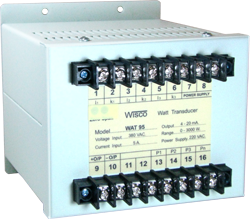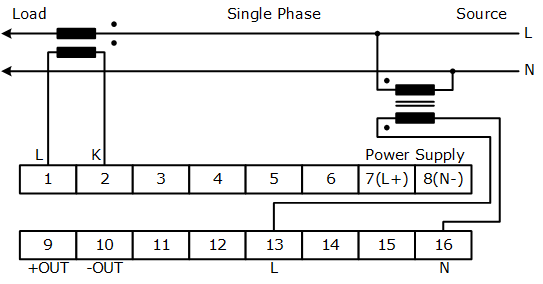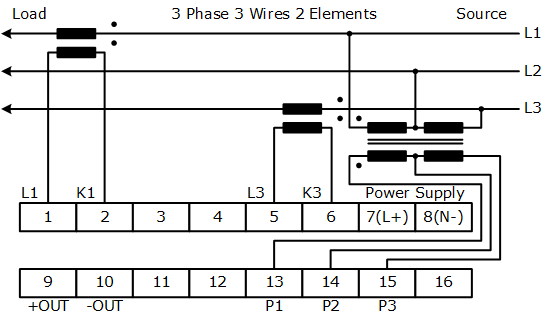# WAT95: Watt Transducer Vietnam

 - High accuracy - Long term stability - Time-Division Multiplication principle

Watt Transducers WAT95 are used to convert single or three phaseunbalance load active power into a proportional load independent DC. Output. Based fully on electronic Time-Division Multiplication measuring principle, the true power of a network with any power factor range are linearly produced by Watt Transducer output.Calibration watt of rated output Connection V=110V V=220V V=380V I=1A I=5A I=1A I=5A I=1A I=5A Single Phase 100W 500W 200W 1000W - - 3phase,3wire 200W 1000W 400W 2000W 600W 3000W 3phase,4wire 200W 1000W 400W 2000W 600W 3000W
 Note. V= Line voltage Equation to calculated measuring range Measuring range = Calibration watt x PT ratio x CT ratio Example 3phase,3wire, PT 220V/110V, CT 250/5A, Calibration watt = 1000W Measuring range = 1000 x 220/110 x 250/5 = 100 KW.